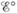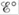# Table 17-1 lists common half-reactions along with the standard reduction potential associated with each half-reaction. These standard reduction potentials are all relative to some standard. What is the standard (zero point)? lf is positive for a half-reaction, what does it mean? If is negative for a half-reaction, what does it mean? Which species in Table 17-1 is most easily reduced? Least easily reduced? The reverse of the half-reactions in Table 17-1 are the oxidation half-reactions. How are standard oxidation potentials determined? In Table 17-1, which species is the best reducing agent? The worst reducing agent? To determine the standard cell potential for a redox reaction, the standard reduction potential is added to the standard oxidation potential. What must be true about this sum if the cell is to be spontaneous (produce a galvanic cell)? Standard reduction and oxidation potentials are intensive. What does this mean? Summarize how line notation is used to describe galvanic cells.### Chemistry: An Atoms First Approach

2nd Edition
Steven S. Zumdahl + 1 other
Publisher: Cengage Learning
ISBN: 9781305079243

#### Solutions

Chapter
Section### Chemistry: An Atoms First Approach

2nd Edition
Steven S. Zumdahl + 1 other
Publisher: Cengage Learning
ISBN: 9781305079243
Chapter 17, Problem 3RQ
Textbook Problem
5 views

## Table 17-1 lists common half-reactions along with the standard reduction potential associated with each half-reaction. These standard reduction potentials are all relative to some standard. What is the standard (zero point)? lfis positive for a half-reaction, what does it mean? Ifis negative for a half-reaction, what does it mean? Which species in Table 17-1 is most easily reduced? Least easily reduced? The reverse of the half-reactions in Table 17-1 are the oxidation half-reactions. How are standard oxidation potentials determined? In Table 17-1, which species is the best reducing agent? The worst reducing agent?To determine the standard cell potential for a redox reaction, the standard reduction potential is added to the standard oxidation potential. What must be true about this sum if the cell is to be spontaneous (produce a galvanic cell)? Standard reduction and oxidation potentials are intensive. What does this mean? Summarize how line notation is used to describe galvanic cells.

Interpretation Introduction

Interpretation:

The table for common half-reactions along with their standard reduction potential with reference to some standard is given. The significance of E° for the half-reactions is to be stated.

Concept introduction:

The standard reduction potential for different species is determined with respect to a standard hydrogen electrode that is used as a reference. Higher the value of standard reduction potential, easily the species gets reduced.

To give: The definition of standard (zero point), significance of positive and negative values of E° ; the easily reduced and oxidized species, the determination of standard oxidation potential; the best and the worst reducing agent; the condition for the cell reaction to be spontaneous; significance of intensive property of standard reduction and oxidation potential and the representation of line notation for a cell reaction.

### Explanation of Solution

When the hydrogen gas is bubbled through the platinum electrode dipped in 1M HCl at the room temperature is called the standard hydrogen electrode and the potential of this electrode is determined to be zero.

As the electrode potential of hydrogen is zero, therefore the oxidation or reduction potential of any chemical species is determined using the hydrogen electrode as the reference.

If E° is positive for a half-reaction, it means the species can accept electron and gets reduced by the species having lower reduction potential, while the negative value for E° shows that the species can release electrons and get easily oxidized.

The value of E° determines the reduction tendency of species. In the given table, the half-reactions are arranged on the basis of their reduction potential. Higher the value of reduction potential greater is the tendency of species to get reduced, while the lower value determines that species is not easily reduced. As the value of standard reduction potential approaches negative values, it means the species has the tendency to oxidize easily.

Referring to the Table- 171 , the species which gets most easily reduced is F2 , while the species which gets least easily reduced is Li .

F2 has the highest value of the standard reduction potential which means it can easily accept electron and gets easily reduced, while Li has the least value of standard reduction potential which shows that it has the least tendency to accept electrons . Li has more tendency to oxidize than to get reduced.

The standard oxidation potential can be determined by writing the reduction half-reaction in reverse order and multiplying the standard reduction potential with minus sign.

When the reaction is written in the form of oxidation reaction, the value of standard potential reduction potential is written with minus sign that gives the standard oxidation potential for the oxidation half-reaction.

Table 171 shows that Li is the best reducing agent, while F2 is the worst reducing agent.

As Li has the least value of standard reduction potential, it means it has more tendency to undergo oxidation reaction rather than the reduction. Therefore, it will lose electrons and itself get oxidized and will reduce the other species. On the other hand, F2 has the highest value of the standard reduction potential

### Still sussing out bartleby?

Check out a sample textbook solution.

See a sample solution

#### The Solution to Your Study Problems

Bartleby provides explanations to thousands of textbook problems written by our experts, many with advanced degrees!

Get Started

Find more solutions based on key concepts
The Academy of Nutrition and Dietetics recommends fluoride-free water for the U.S. population. T F

Nutrition: Concepts and Controversies - Standalone book (MindTap Course List)

What is population genetics?

Human Heredity: Principles and Issues (MindTap Course List)

What is a hot spot? Where can you go to see hot-spot activity?

Oceanography: An Invitation To Marine Science, Loose-leaf Versin

If a loop of chain is spun at high speed, it can roll along the ground like a circular hoop without collapsing....

Physics for Scientists and Engineers, Technology Update (No access codes included)